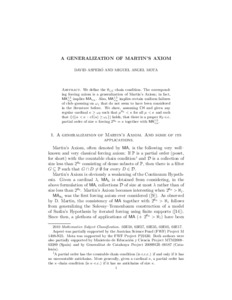# A generalization of Martin's Axiom

Aspero, David and Mota, Miguel Angel (2015) A generalization of Martin's Axiom. Israel Journal of Mathematics, 210 (1). pp. 193-231. ISSN 0021-2172Preview PDF (generalizationma(revised)-1) - Draft Version Download (543kB) | Preview

## Abstract

We define the $$\aleph_{1.5}$$-chain condition. The corresponding forcing axiom is a generalization of Martin's Axiom; in fact, $$MA^{1.5}_{<\kappa}$$ implies $$MA_{<\kappa}$$. Also, $$MA^{1.5}_{<\kappa}$$ implies certain uniform failures of club-guessing on $$\omega_1$$ that do not seem to have been considered in the literature before. We show, assuming CH and given any regular cardinal $$\kappa\geq\omega_2$$ such that $$\mu^{\aleph_0}< \kappa$$ for all $$\mu < \kappa$$ and such that $$\diamondsuit(\{\alpha<\kappa\,:\, cf(\alpha)\geq\omega_1\})$$ holds, that there is a proper $$\aleph_2$$-c.c. partial order of size $$\kappa$$ forcing $$2^{\aleph_0}=\kappa$$ together with $$MA^{1.5}_{<\kappa}$$.

Item Type: Article Faculty of Science > School of Mathematics Pure Connector 19 Dec 2015 07:04 24 Oct 2022 06:30 https://ueaeprints.uea.ac.uk/id/eprint/55751 10.1007/s11856-015-1250-0View Item